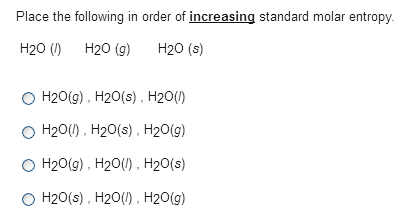Chemistry Practice Problems Entropy Practice Problems Solution: Place the following in order of increasing standar...

🤓 Based on our data, we think this question is relevant for Professor Wingrave's class at UD.

# Solution: Place the following in order of increasing standard molar entropy.H2O(l) H2O(g) H2O(s) a. H2O(g), H2O(s), H2O(l) b. H2O(l), H2O(s), H2O(g) c. H2O(g), H2O(l), H2O(s) d. H2O(s), H2O(l), H2O(g)

###### Problem

Place the following in order of increasing standard molar entropy.

H2O(l) H2O(g) H2O(s)

a. H2O(g), H2O(s), H2O(l)

b. H2O(l), H2O(s), H2O(g)

c. H2O(g), H2O(l), H2O(s)

d. H2O(s), H2O(l), H2O(g)View Complete Written Solution

Entropy

Entropy

#### Q. Sodium reacts violently with water according to the equation: 2 Na(s) + 2 H2O(l) →2NaOH(aq) + H2(g) The resulting solution has a higher temperature th...

Solved • Mon Jul 30 2018 16:45:25 GMT-0400 (EDT)

Entropy

#### Q. Entropy is a measure of a. free energy. b. the heat of a reaction. c. the rate of a reaction. d. molecular randomness.

Solved • Mon Jul 30 2018 16:25:15 GMT-0400 (EDT)

Entropy

#### Q. Which provides the greatest decrease in entropy?

Solved • Mon Jul 30 2018 13:41:57 GMT-0400 (EDT)

Entropy

#### Q. The entropy of 1 mole of N2 (g) is greater than the entropy of 1 mole of N2(aq).a. Trueb. False

Solved • Tue Jul 24 2018 10:26:53 GMT-0400 (EDT)